Kai Ho

•Activity

Kai Ho

•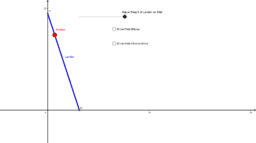Activity

Kai Ho

•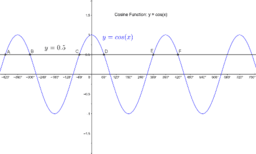SM11 2.1.1 Cos x = k graph in deg

Activity

Kai Ho

•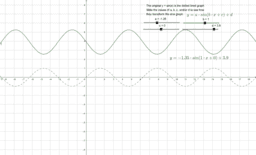MM2.1.2 the sine graph

Activity

Kai Ho

•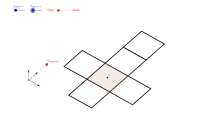Cuboides 2

Activity

Kai Ho

•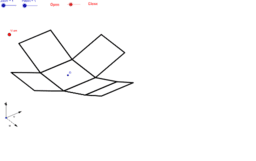Copy of 11 paterns of the cube

Activity

Kai Ho

•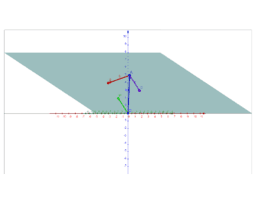Copy of Vector Equation of a Plane 3D

Activity

Kai Ho

•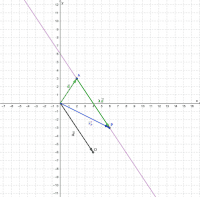Vector, parametric & Cartesian equations of a line (2D)

Activity

Kai Ho

•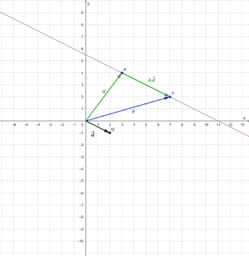Vector & Cartesian equation of a line (2D)

Activity

Kai Ho

•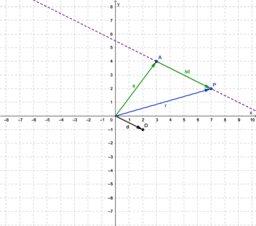Copy of Vector equation of a line (2D)

Activity

Kai Ho

•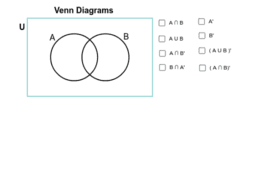Copy of Venn Diagrams

Activity

Kai Ho

•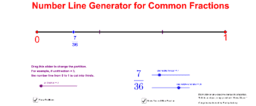Copy of Making Number Lines with Common Fractions (for Teachers)

Activity

Kai Ho

•Copy of Making Number Lines with Common Fractions (for Teachers)

Activity

Kai Ho

•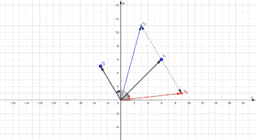Copy of Complex number arithmetic and geometry

Activity

Kai Ho

•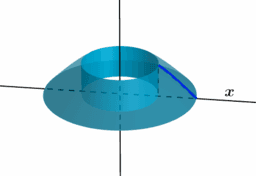Copy of Volume by Shell Graphic

Activity

Kai Ho

•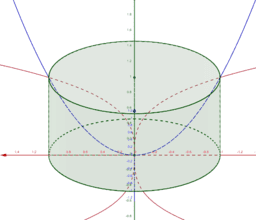Copy of volume by shells

Activity

Kai Ho

•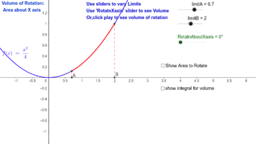Copy of Integration and Volume of solids of rotation

Activity

Kai Ho

•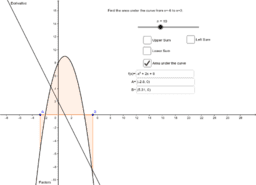Copy of Area Under a Curve

Activity

Kai Ho

•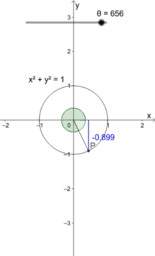Copy of The unit circle and the graphs of sin, cos & tan

Activity

Kai Ho

•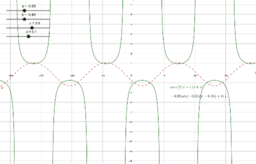2.1.4 secant function

Activity

Kai Ho

•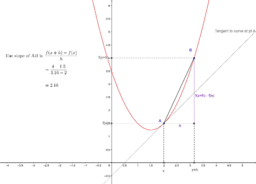Derivative lim process secant line

Activity

Kai Ho

•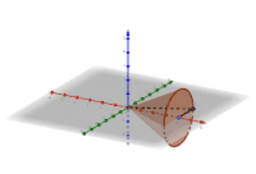SM12_4.1_Cone

Activity

Kai Ho

•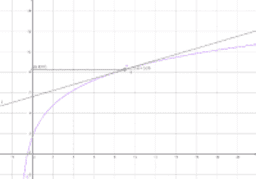Tangent line to demo f'' < 0

Activity

Kai Ho

•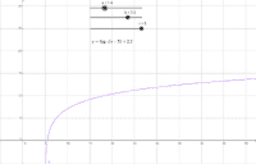Activity

Kai Ho